Most Affordable JEE | NEET | 8,9,10 Preparation by Kota's Top IITian Doctor Faculties

# Chapter 2 - Polynomial of Class 10th - NCERT Solutions

Class 10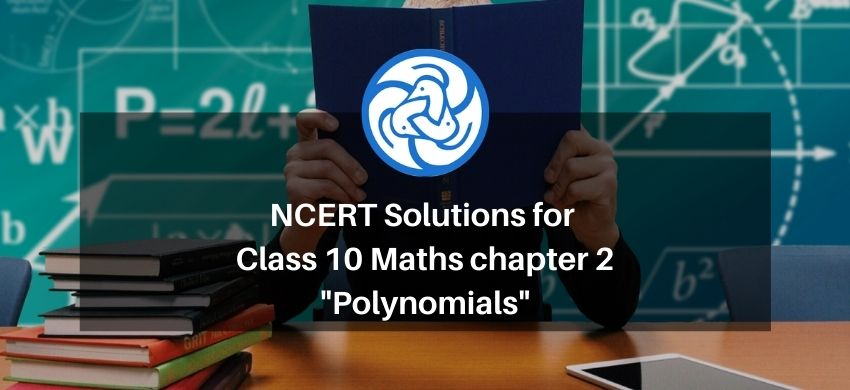`

Chapter 2 Polynomials of Class 10th - NCERT Solutions , provide a comprehensive and step-by-step guide to understanding the basics of polynomials. In this chapter, students will learn about the different types of polynomials, including linear, quadratic, and cubic polynomials. The solutions cover all the topics related to polynomials, including factorization of polynomials, finding zeros of a polynomial, and using the remainder theorem.

The solutions are designed to help students improve their problem-solving skills and understand the concepts in a simple and straightforward manner. With the help of Chapter 2 Polynomial of Class 10th - NCERT Solutions, students can practice and revise the concepts regularly, ensuring that they have a strong foundation in the subject.

Overall, the  for  Chapter 2 Polynomials Class 10 Maths NCERT Solutions, are an excellent resource for students to enhance their knowledge and understanding of polynomials.

If you want to learn and understand class 10 Maths chapter 2 "Polynomials" in an easy way then you can use these solutions PDF.

NCERT Solutions helps students to Practice important concepts of subjects easily. Class 10 Maths solutions provide detailed explanations of all the NCERT exercise questions that students can use to clear their doubts instantly.

If you want to score high in your class 10 Maths Exam then it is very important for you to have a good knowledge of all the important topics, so to learn and practice those topics you can use eSaral NCERT Solutions.

So, without wasting more time Let’s start.

### Download The PDF of NCERT Solutions for Class 10 Maths Chapter 2 "Polynomials"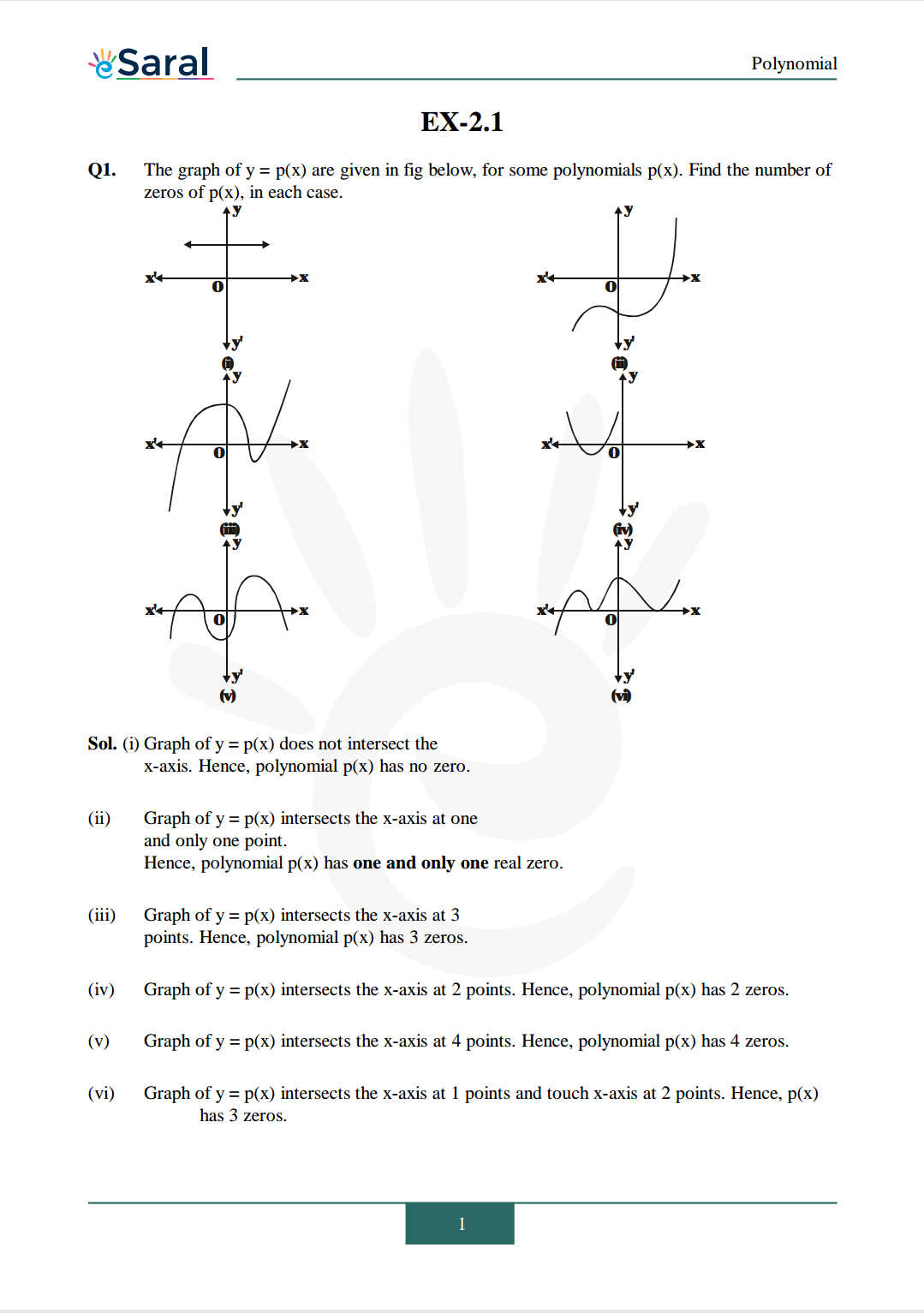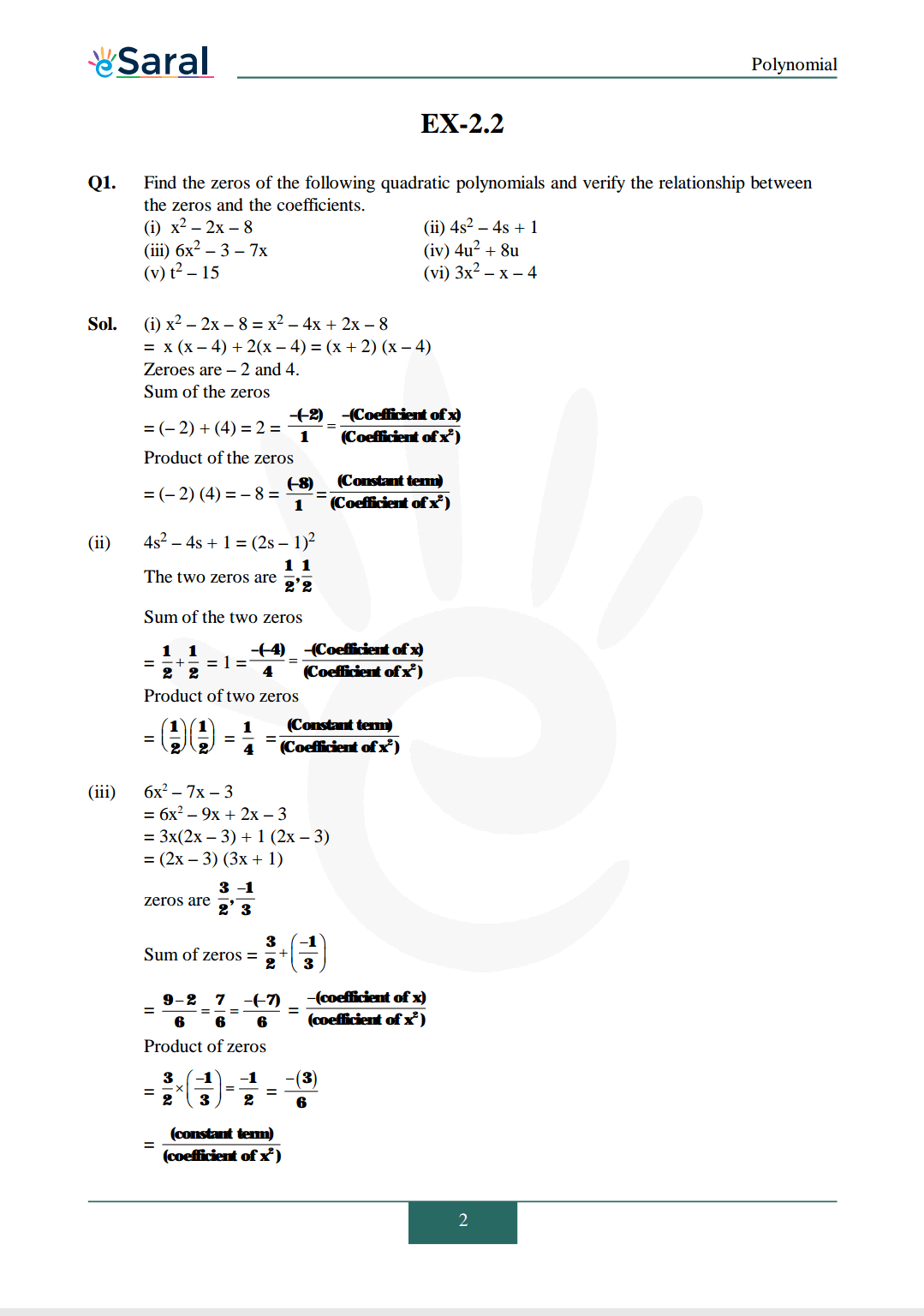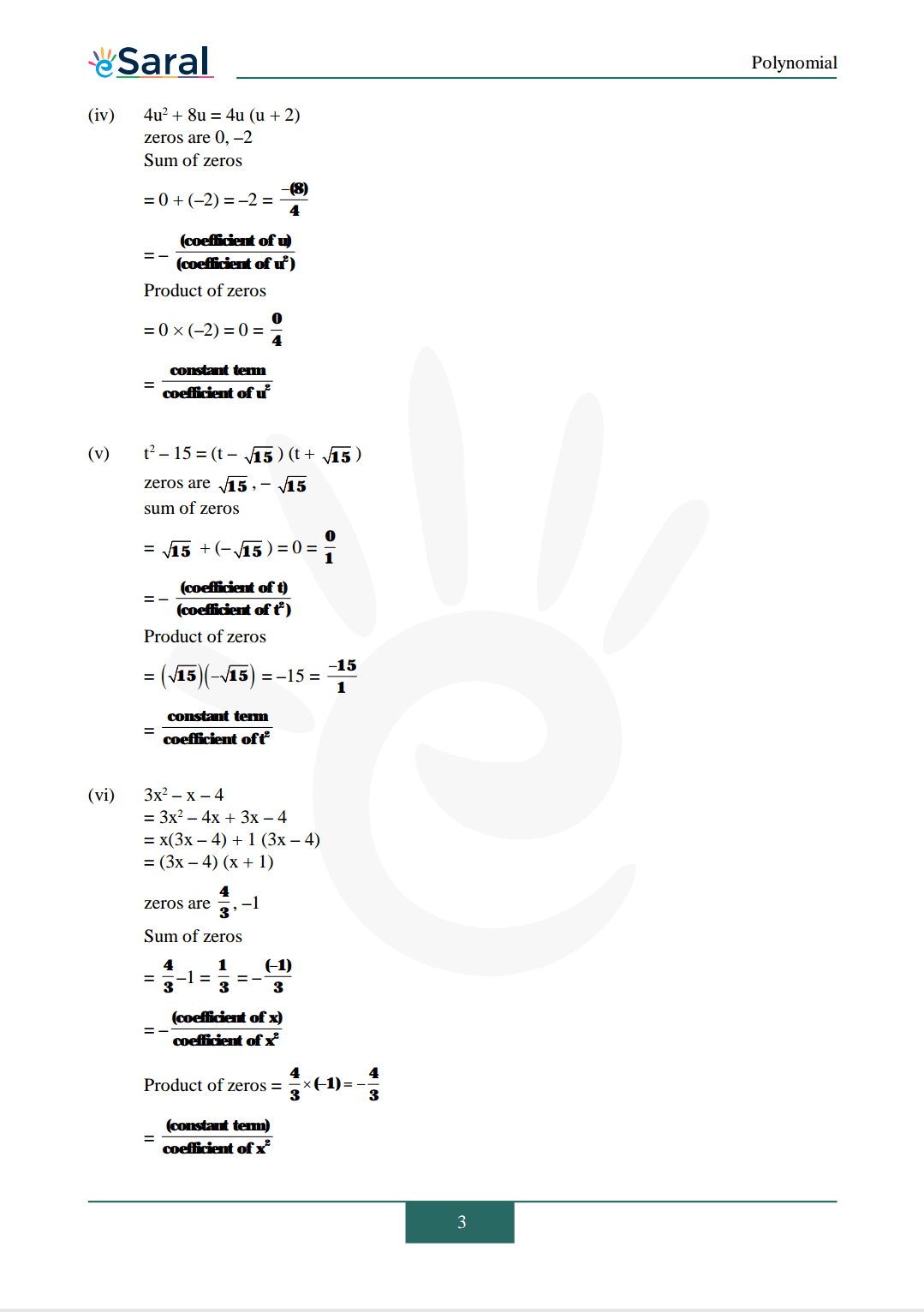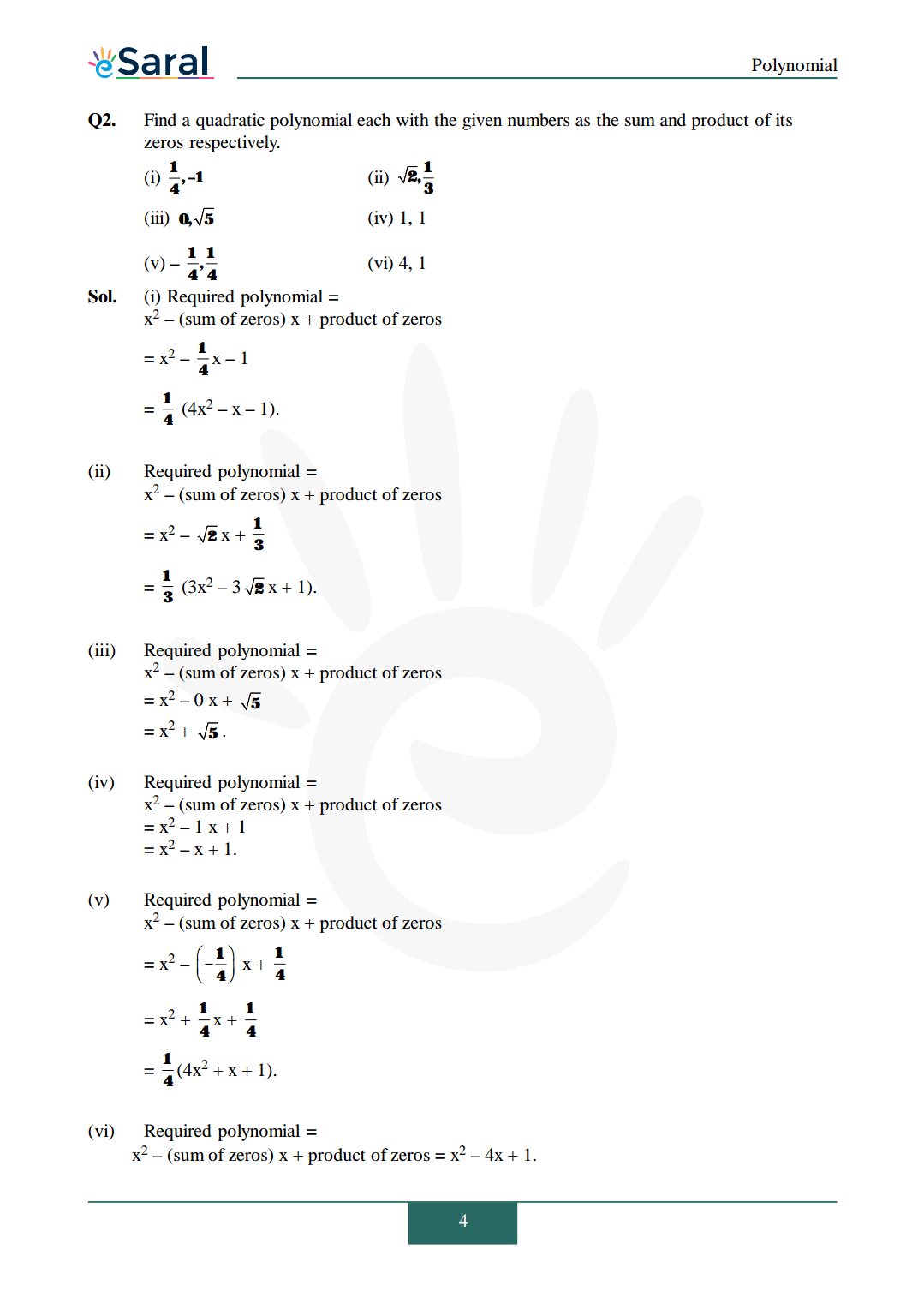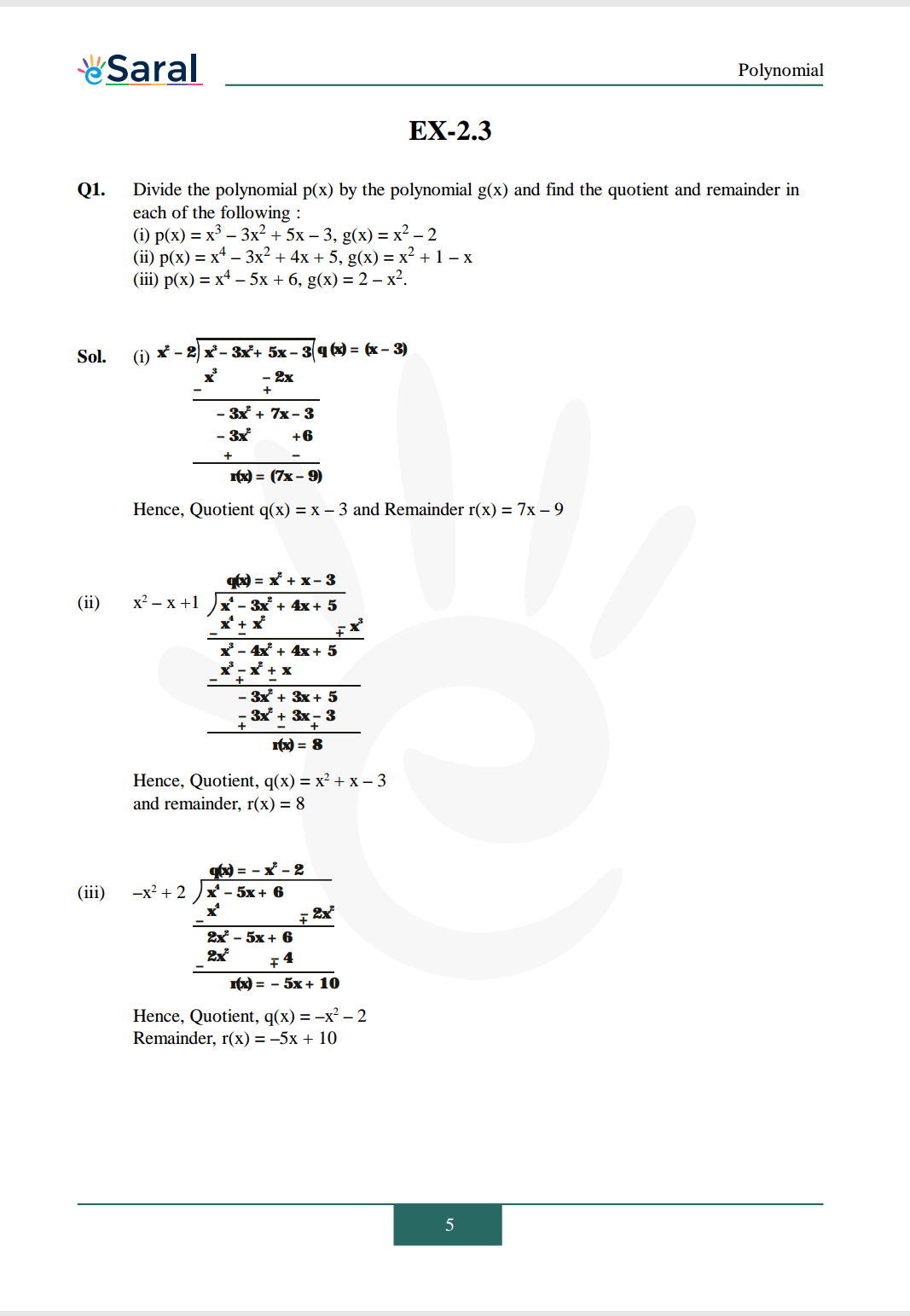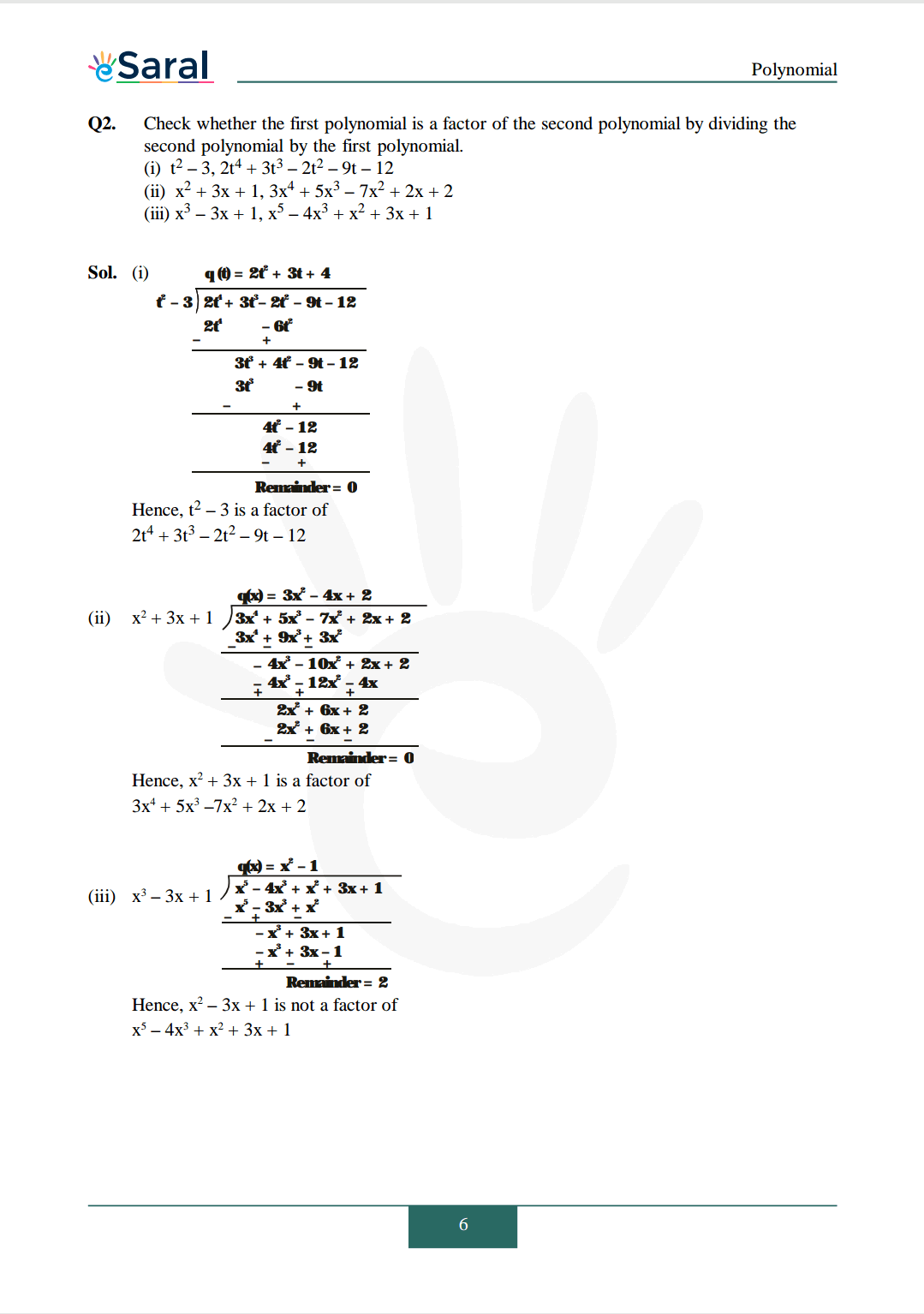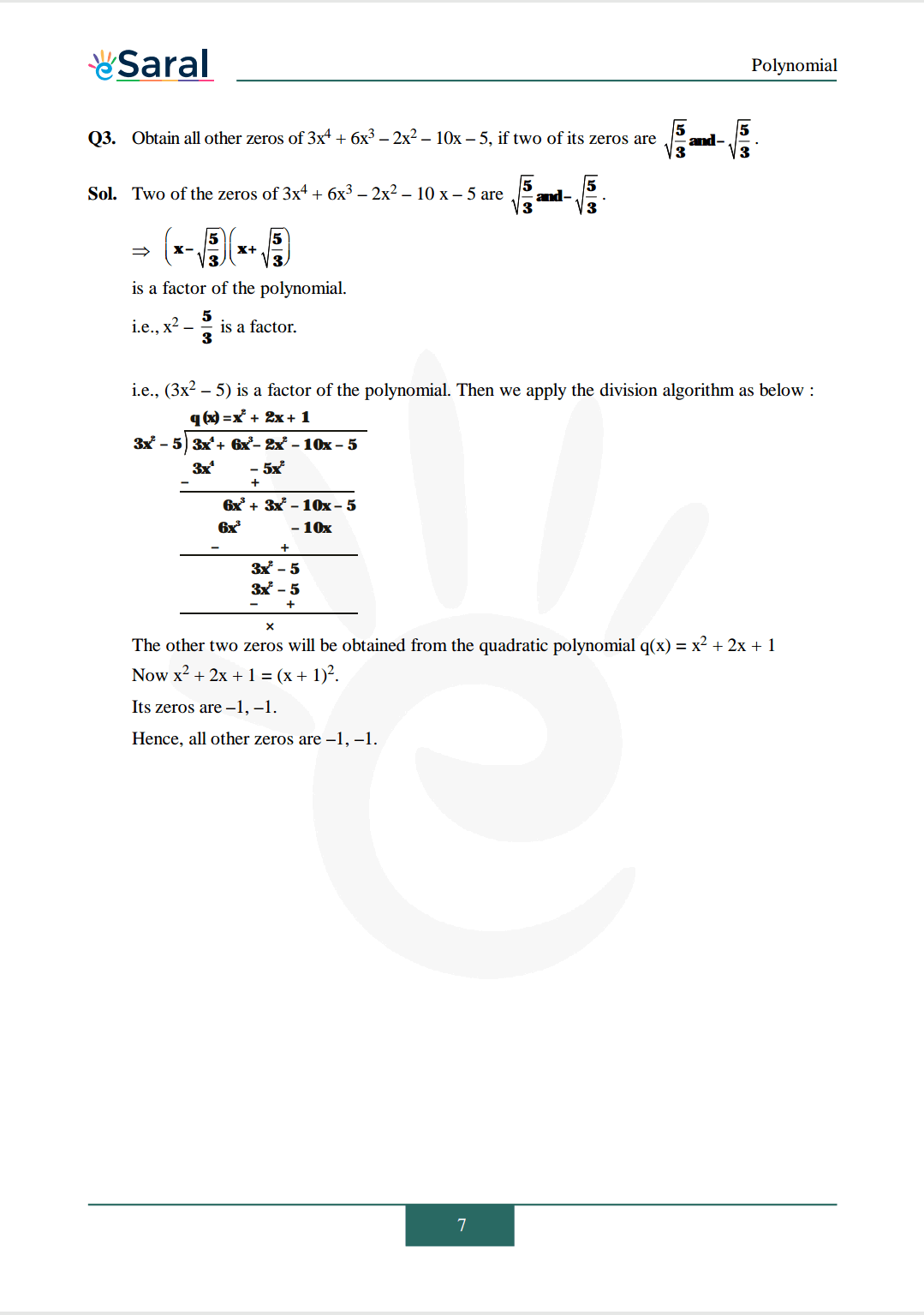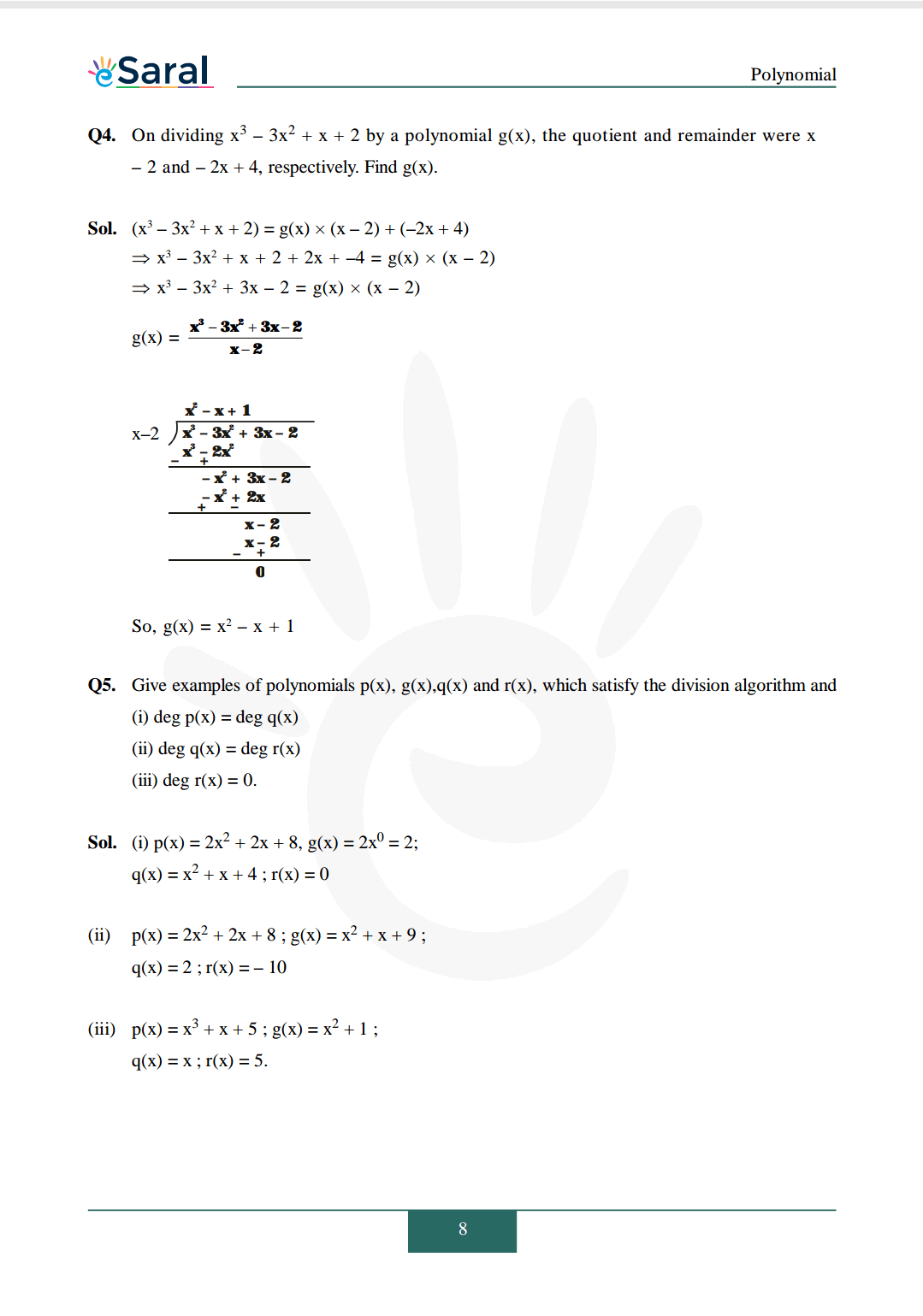Download The PDF of NCERT Solutions for Class 10 Maths Chapter 2 "Polynomials"

## What is Polynomial ?

A polynomial is an algebraic expression that contains variables, coefficients, and exponents. It is a sum of terms, where each term has a variable raised to a non-negative integer power. The degree of a polynomial is the highest power of its variable.

### Types of Polynomial.

There are several types of polynomials, including linear, quadratic, and cubic polynomials.

A linear polynomial is a polynomial of degree 1, which means that it has only one variable raised to the first power. Its general form is ax + b, where a and b are constants.

A quadratic polynomial is a polynomial of degree 2, which means that it has one variable raised to the second power. Its general form is ax^2 + bx + c, where a, b, and c are constants.

A cubic polynomial is a polynomial of degree 3, which means that it has one variable raised to the third power. Its general form is ax^3 + bx^2 + cx + d, where a, b, c, and d are constants.

Polynomials can have higher degrees as well, and their forms become more complex as the degree increases.

## Tips and Tricks for solving problems related to Chapter 2 - Polynomial of Class 10th - NCERT Solutions

1. Understand the problem: Before starting to solve a problem, make sure you understand the given problem statement and the type of polynomial involved.

2. Identify the degree of the polynomial: Determine the degree of the polynomial involved, as it will help in understanding the type of polynomial and the methods to be used for solving it.

3. Factorize the polynomial: Factorizing the polynomial can make it easier to find the zeros of the polynomial and simplify the expression.

4. Use the remainder theorem: The remainder theorem is a useful technique to find the value of a polynomial at a given point. It states that if the polynomial f(x) is divided by (x - a), then the remainder is equal to f(a).

5. Practice solving different types of problems: Practice is key to mastering any subject, including polynomials. Solve a variety of problems related to polynomials, including finding zeros, factorization, and evaluation of polynomials.

6. Check your answers: After solving a problem, always check your answer to ensure that it satisfies the given conditions and that the calculation is correct.

By following these tips and tricks, you can improve your problem-solving skills and gain a better understanding of polynomials.

### Common mistakes to avoid while solving problems related to Polynomials:

1. Misidentifying the degree of the polynomial: It is essential to correctly identify the degree of the polynomial, as it will determine the methods used to solve it.

2. Forgetting to simplify the expression: While solving problems involving polynomials, it is important to simplify the expressions as much as possible to make the calculations easier.

3. Making arithmetic errors: Polynomials involve a lot of arithmetic calculations, and it is common to make errors while performing calculations such as addition, subtraction, multiplication, and division.

4. Misinterpreting the problem: Misunderstanding the problem statement or making incorrect assumptions can lead to incorrect solutions.

5. Forgetting to check your answers: It is important to check your answers after solving the problem to ensure that it satisfies the given conditions and that the calculation is correct.

6. Using incorrect formulas or theorems: It is important to use the correct formulas and theorems while solving problems related to polynomials, as using incorrect formulas or theorems can lead to incorrect solutions.

By being aware of these common mistakes and taking steps toimg avoid them, you can improve your problem-solving skills and achieve more accurate solutions while working with polynomials

Class 10 Maths Quick Revision Notes.

Class 10 Maths Book

Class 10 Maths Exemplar

If you have any Confusion related to Chapter 2 Polynomial of Class 10th - NCERT Solutions then feel free to ask in the comments section down below.

To watch Free Learning Videos on Class 10 by Kota’s top Faculties Install the eSaral App

## Q.1.Is it necessary to solve each problem provided in the Chapter 2 - Polynomial of Class 10th - NCERT Solutions ?

A1.Yes. Because these questions are important from an exam perspective. These questions are solved by experts to help the students to crack each exercise very easily. These solutions help students to familiarize themselves with the polynomials

## Q2.List out the concepts covered in NCERT Solutions for Class 10 Maths Polynomials.

A2.The concepts covered in NCERT Solutions for Class 10 Maths Polynomials are introduction to polynomials, geometrical meaning of the zeros of polynomial, relationship between zeros and coefficients of a polynomial and division algorithm for polynomials. By learning these concepts students will be able to solve questions on polynomials

## Q3.How many exercises are there in Class 10 Polynomials?

A3.The important topics covered in NCERT solutions class 10 maths chapter 2 are the definition of a zero of a polynomial, degrees of a polynomial, and the division algorithm of polynomials. Polynomials Chapter 2 is very diverse and helps the students understand concepts such as parabolas, graphs, and the geometric meaning of zeros of the polynomial.